# REPT Function

Repeats characters a given number of times

## What is the REPT Function?

The REPT Function is categorized under Excel Logical functions. The function will repeat characters a given number of times.

In financial analysis, we can use the REPT function to fill a cell or pad values to a certain length. We can also build histograms, a chart commonly used in financial modeling, using the function by translating values directly into a certain number of (repeated) characters.

### Formula

=REPT(text, number_times)

The REPT function uses the following arguments:

1. Text (required argument) – This is the text we want to repeat.
2. Number_times (required argument) – This is the positive number that specifies the number of times to repeat text.

### How to use the REPT Function in Excel?

As a worksheet function, the REPT function can be entered as part of a formula in a cell of a worksheet. To understand the uses of the function, let us consider a few examples:

#### Example 1

Suppose we are given the data below: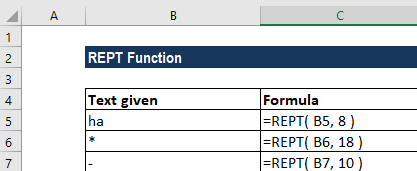The results in Excel are shown in the screenshot below: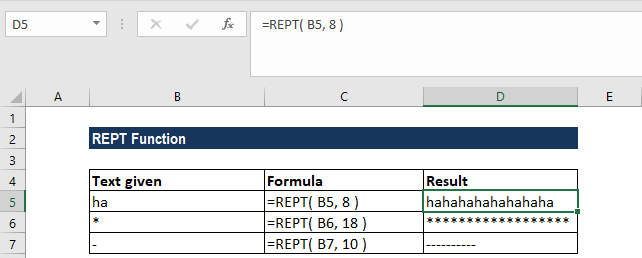#### Example 2

To create a simple in-cell histogram, we can use a formula based on the REPT function. It can be useful when we want to avoid the complexity of creating a separate chart.

Using the data below: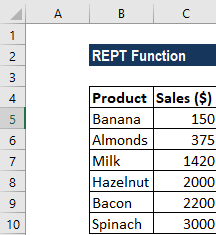We can use REPT to repeat any character(s) we want. In the example, we used the CHAR function to output a character with a code of 110. The character, when formatted with the Wingdings font, will output a solid square. The formula to use will be: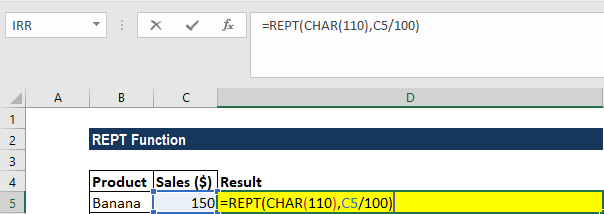The results we get in Wingdings font are shown below: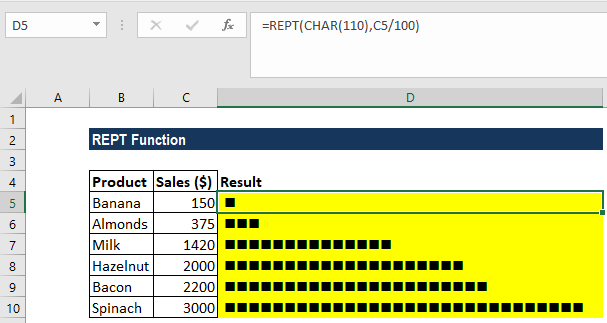### A few notes about the REPT Function:

1. #VALUE! error – Occurs if the result of the REPT function is more than 32,767 characters long.
2. If number_times is not an integer, it is truncated.
3. If number_times is 0 (zero), REPT returns “” (empty text).

Thanks for reading CFI’s guide to important Excel functions! By taking the time to learn and master these functions, you’ll significantly speed up your financial analysis. To learn more, check out these additional CFI resources:

• Excel Functions for Finance